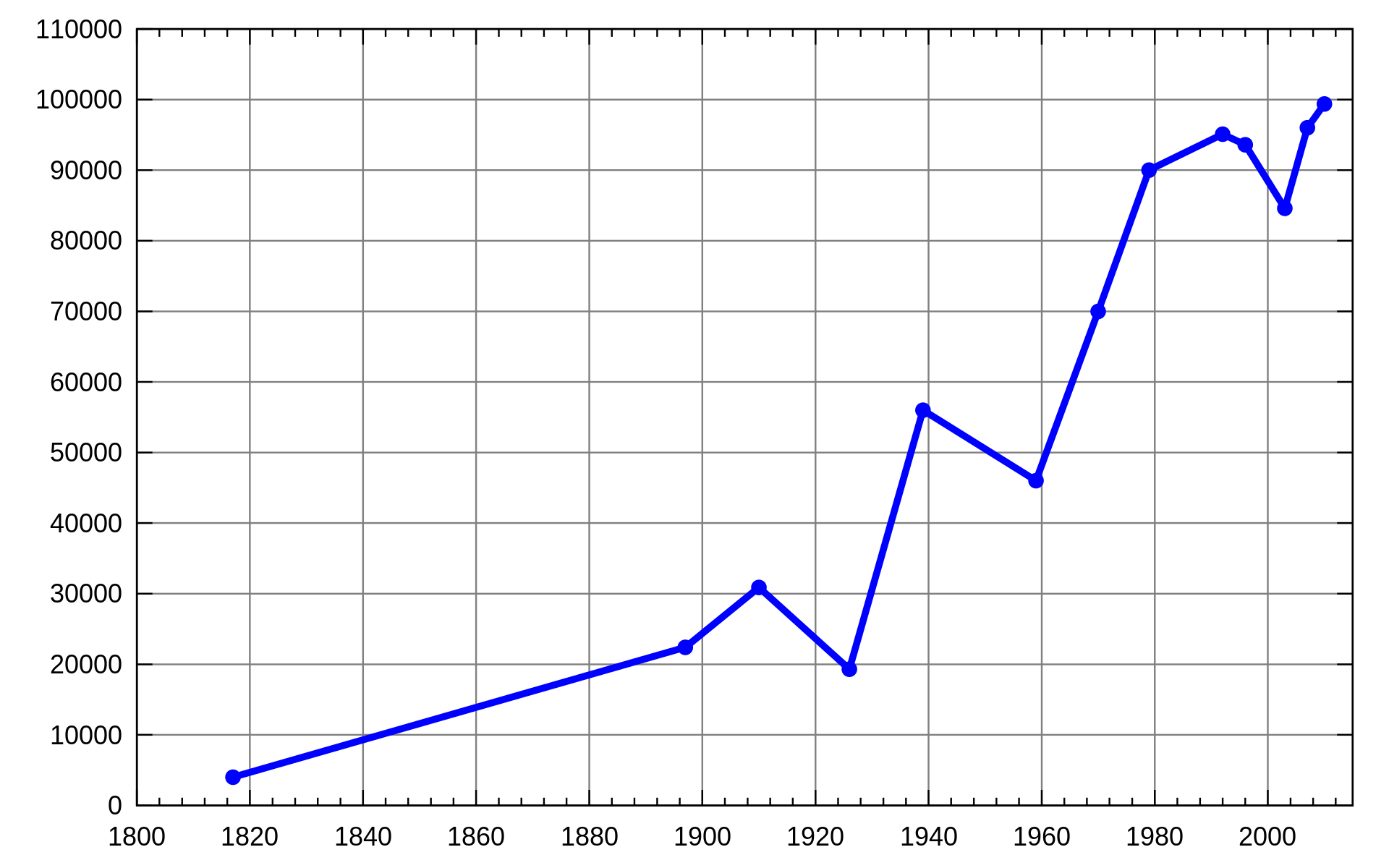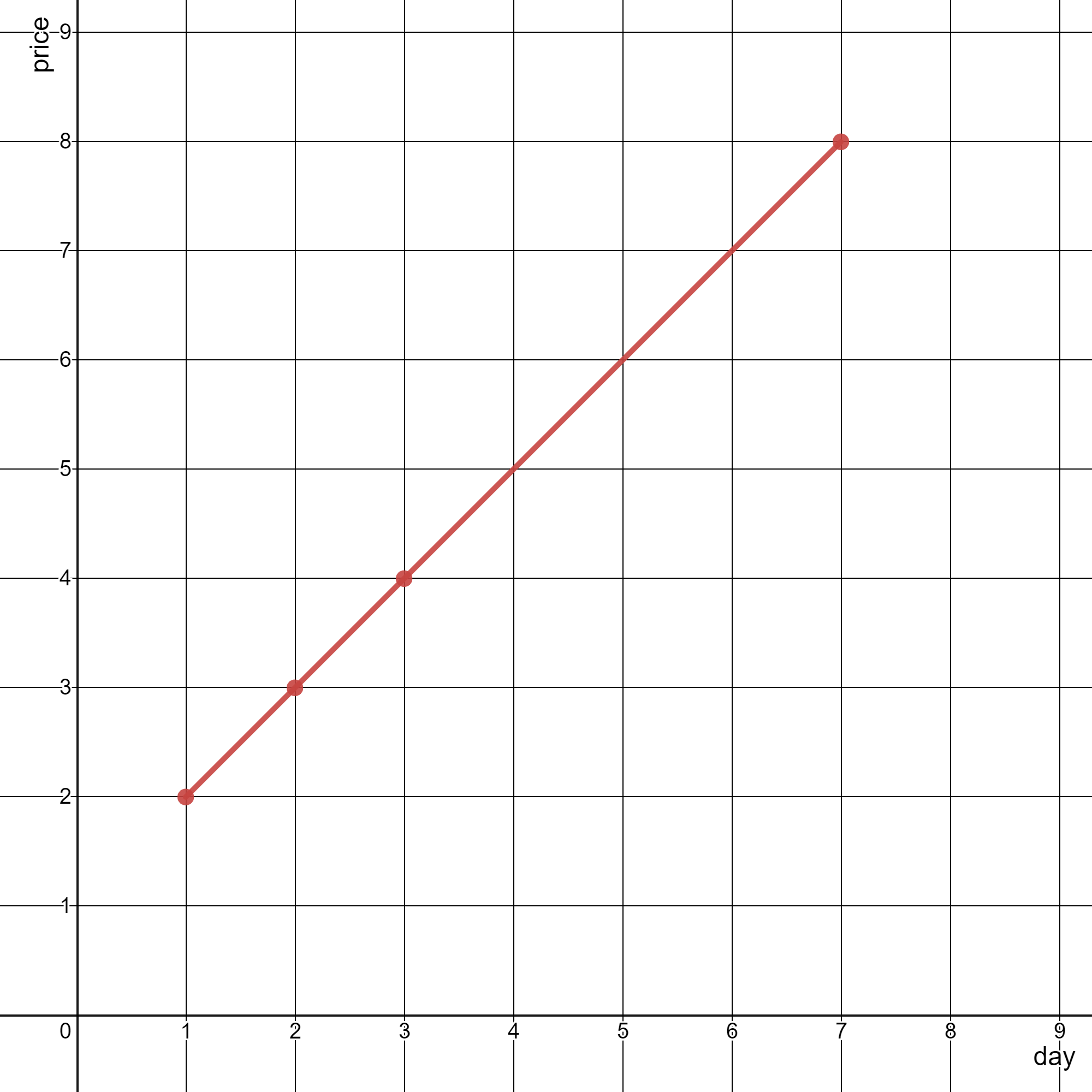##### Welcome to Subscribe On Youtube

Formatted question description: https://leetcode.ca/all/2280.html

# 2280. Minimum Lines to Represent a Line Chart

• Difficulty: Medium.
• Related Topics: Array, Math, Geometry, Sorting, Number Theory.
• Similar Questions: Max Points on a Line, Minimum Number of Lines to Cover Points.

## Problem

You are given a 2D integer array stockPrices where stockPrices[i] = [dayi, pricei] indicates the price of the stock on day dayi is pricei. A line chart is created from the array by plotting the points on an XY plane with the X-axis representing the day and the Y-axis representing the price and connecting adjacent points. One such example is shown below:Return the **minimum number of lines needed to represent the line chart**.

Example 1:Input: stockPrices = [[1,7],[2,6],[3,5],[4,4],[5,4],[6,3],[7,2],[8,1]]
Output: 3
Explanation:
The diagram above represents the input, with the X-axis representing the day and Y-axis representing the price.
The following 3 lines can be drawn to represent the line chart:
- Line 1 (in red) from (1,7) to (4,4) passing through (1,7), (2,6), (3,5), and (4,4).
- Line 2 (in blue) from (4,4) to (5,4).
- Line 3 (in green) from (5,4) to (8,1) passing through (5,4), (6,3), (7,2), and (8,1).
It can be shown that it is not possible to represent the line chart using less than 3 lines.


Example 2:Input: stockPrices = [[3,4],[1,2],[7,8],[2,3]]
Output: 1
Explanation:
As shown in the diagram above, the line chart can be represented with a single line.


Constraints:

• 1 <= stockPrices.length <= 105

• stockPrices[i].length == 2

• 1 <= dayi, pricei <= 109

• All dayi are distinct.

## Solution (Java, C++, Python)

• class Solution {
public int minimumLines(int[][] stockPrices) {
if (stockPrices.length == 1) {
return 0;
}
Arrays.sort(stockPrices, (a, b) -> a - b);
// multiply with 1.0 to make it double and multiply with 100 for making it big so that
// difference won't come out to be very less and after division it become 0.
// failing for one of the case without multiply 100
double lastSlope =
(stockPrices - stockPrices)
* 100
/ ((stockPrices - stockPrices) * 1.0);
int ans = 1;
for (int i = 2; i < stockPrices.length; i++) {
double curSlope =
(stockPrices[i] - stockPrices[i - 1])
* 100
/ ((stockPrices[i] - stockPrices[i - 1]) * 1.0);
if (lastSlope != curSlope) {
lastSlope = curSlope;
ans++;
}
}
return ans;
}
}

############

class Solution {
public int minimumLines(int[][] stockPrices) {
Arrays.sort(stockPrices, (a, b) -> a - b);
int dx = 0, dy = 1;
int ans = 0;
for (int i = 1; i < stockPrices.length; ++i) {
int x = stockPrices[i - 1], y = stockPrices[i - 1];
int x1 = stockPrices[i], y1 = stockPrices[i];
int dx1 = x1 - x, dy1 = y1 - y;
if (dy * dx1 != dx * dy1) {
++ans;
}
dx = dx1;
dy = dy1;
}
return ans;
}
}

• class Solution:
def minimumLines(self, stockPrices: List[List[int]]) -> int:
stockPrices.sort()
dx, dy = 0, 1
ans = 0
for (x, y), (x1, y1) in pairwise(stockPrices):
dx1, dy1 = x1 - x, y1 - y
if dy * dx1 != dx * dy1:
ans += 1
dx, dy = dx1, dy1
return ans

############

# 2280. Minimum Lines to Represent a Line Chart
# https://leetcode.com/problems/minimum-lines-to-represent-a-line-chart/

class Solution:
def minimumLines(self, stock: List[List[int]]) -> int:
n = len(stock)

if n == 1: return 0

stock.sort(key = lambda x: x)
dx = stock - stock
dy = stock - stock

res = 1

for index in range(2, n):
x, y = stock[index]

dx1 = stock[index - 1] - stock[index]
dy1 = stock[index - 1] - stock[index]

if dx1 * dy != dx * dy1:
res += 1
dx = dx1
dy = dy1

return res


• class Solution {
public:
int minimumLines(vector<vector<int>>& stockPrices) {
sort(stockPrices.begin(), stockPrices.end());
int dx = 0, dy = 1;
int ans = 0;
for (int i = 1; i < stockPrices.size(); ++i) {
int x = stockPrices[i - 1], y = stockPrices[i - 1];
int x1 = stockPrices[i], y1 = stockPrices[i];
int dx1 = x1 - x, dy1 = y1 - y;
if ((long long) dy * dx1 != (long long) dx * dy1) ++ans;
dx = dx1;
dy = dy1;
}
return ans;
}
};

• func minimumLines(stockPrices [][]int) int {
ans := 0
sort.Slice(stockPrices, func(i, j int) bool { return stockPrices[i] < stockPrices[j] })
for i, dx, dy := 1, 0, 1; i < len(stockPrices); i++ {
x, y := stockPrices[i-1], stockPrices[i-1]
x1, y1 := stockPrices[i], stockPrices[i]
dx1, dy1 := x1-x, y1-y
if dy*dx1 != dx*dy1 {
ans++
}
dx, dy = dx1, dy1
}
return ans
}

• function minimumLines(stockPrices: number[][]): number {
const n = stockPrices.length;
stockPrices.sort((a, b) => a - b);
let ans = 0;
let pre = [BigInt(0), BigInt(0)];
for (let i = 1; i < n; i++) {
const [x1, y1] = stockPrices[i - 1];
const [x2, y2] = stockPrices[i];
const dx = BigInt(x2 - x1),
dy = BigInt(y2 - y1);
if (i == 1 || dx * pre !== dy * pre) ans++;
pre = [dx, dy];
}
return ans;
}



Explain:

nope.

Complexity:

• Time complexity : O(n).
• Space complexity : O(n).